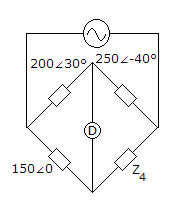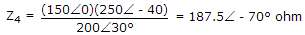# Electronics and Communication Engineering - Measurements and Instrumentation

### Exercise :: Measurements and Instrumentation - Section 1

46.

A dynamometer wattmeter is connected in ac circuit. The measured power is

 A. rms power B. average power C. peak power D. instantaneous power

Explanation:

All wattmeters measure average power over a cycle.

47.

Loss of charge method is suitable for medium resistances.

 A. True B. False

Explanation:

It is suitable for high resistances.

48.

In two wattmeter method of 3 phase power measurement, when does one wattmeter read negative

 A. when power factor is less than 0.5 lagging B. when power factor is greater than 0.5 lagging C. when power factor is less than 0.5 D. when power factor is unity

Explanation:

When p.f. is less than 0.5 (leading or lagging) one of the wattmeters reads negative.

49.

It is required to measure angular position. Out of the transducers

1. Circular potentiometer
2. LVDT
3. E pick up
4. Synchro
The proper devices are

 A. 1, 2, 3, 4 B. 2 and 3 C. 1, 2, 4 D. 1 and 4

Explanation:

LVDT and E pickup are suitable for linear displacement.

50.

In figure the bridge is balanced when Z4 isA. 120∠70° B. 187.5∠ - 10° C. 187.5∠ - 70° D. 333.3∠ - 70°.